Thursday , July 18 2019
Home » Igcse Mathematics Revision Notes » Properties Of A Circle

# Properties Of A Circle

### Circle Definitions: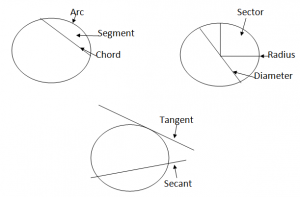### Geometrical Properties of Circle: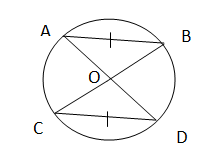If 2 chords in a circle area congruent, then the 2 angles at the centre of the circle are identical.

AB = CD (Equal Chords)

∠ AOB = ∠ COD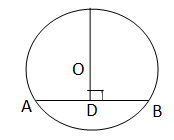The perpendicular bisector of a chord passes through the centre of a circle

E.g.

∠CDA = ∠CDB

=90°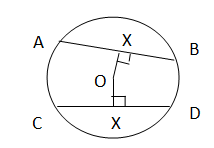2 congurent chords in a circle are of the same distance from the centre of the circle.

E.g.

AB = CD

OX = OY

### Angle Properties Of Cicle:Angle in a semi circle is a right angle.

∠AXB = ∠AYB = 90°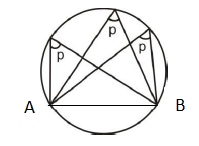Angles in the same segment are equal

∠p = ∠p = ∠p